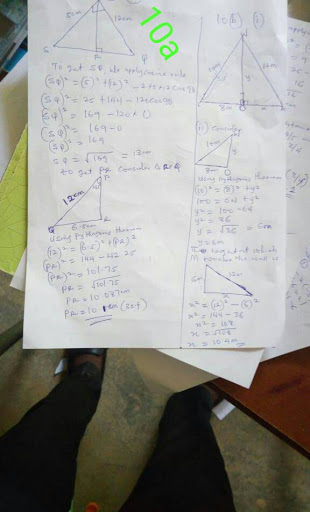# Waec 2018 Mathematics Obj And Theory Answers ExpoMaths Obj
1-10: ACBCDDCBAA
11-20: CDCABCCCAC

(1)
On February 28th 2012, value = (100-30/100) * #900,00.00

= 70/100 * #900,00

= #630,000.00

On february 28th 2013, value = (100-22/1000 * #630,00

= 78/100 8 #630,000

= #491,400

On february 28th 2014, value = 78/100 8 #491,400

=383,292

On february 28th 2015, value = 78/100 * #383,292

= #298,967.76

=============================

(2)
Given that y = 2pxˆ² – p² x – 14

AT (3, 10)

10 = 2p(3)²  – p² (3) – 14

10 = 18p – 3p²  – 14

3p²  – 18p 24 = 0

p²  – 6p 8 = 0

using factor method,

p²  – 2p -4p 8 = 0

p(p-2) – 4(p-2) = 0

(p-4)(p-2) = 0

p-4 = 0 or p-4 = 0

p= 4 or p =2

2b)
The lines must be solved simultenously

3y – 2x = 21 ——- (1)

4y 5x = 5 ——-(2)

using elimination method,

(4)  3y – 2x = 21

(30 4y 5x = 5

12y – 8y = 84 ——— (3)

12y 15x = 15 ——-(4)

equ (4) minus equ(3)

23x = -69

x = -69/23

x = -3

Put this into equation (1)

3y -2(-3) = 21

3y = 6 = 21

3y = 21 -6

3y = 15

y =15/3

y = 5

coordinates of Q is (-3, 5)

3a)
The diagonal = 10.2m and 9.3cm
Using Pythagoras theory
Ac² = 10.2² 9-3²
Ac² = 104.04 86.49
Ac² = 190.53
Ac² = √190.53
Ac² = 13.80

3b)
DRAW THE DIAGRAM
Using Pythagoras theory
5² = 3² x²
x² = 5² - 3²
X²= 25 - 9
X² = √16
X= 4cm
= 4/5
Tan X = opp/adj. = 3/4
5cos x - 4tan x
5(4/5)- 4(3/4)
20/5 - 12/4
4-3= 1
========================

4ai)
sum of angle in a D =180degree
xdegree 90degree 180degree - (3x 15)=180degree
xdegree 90degree 180degree - 3x 15=180degree
-2x=180degree - 255
2x/2= 75/2
x=37.5

4aii)
<RsQ =180 - (3x 15)
<RsQ =180-(3*37.5 15)
=180-(112.5 15)
=180 - 127.5
<RsQ= 52.5degree
=========================

4b)
2N4seven =15Nnine
2*7^2 N*7^1 4*7degree =1*9^2 5*9^1 N*9degree
9*49 N*7 4*1=1*81 5*9 N*1
98 7N 4=81 45 N
7N 102=126 N
7N-N=126-102
6N/6 =24/6
N=4

(10a) Using Pythagoras theorem from SPQ
|SQ|^2 = 12^2 5^2
= 144 25
=169
SQ= sqroot of 169
= 13cm
Sin tita= 5/13 = 0.3846
Tita= Sin^-1(0.3846)
= 22.6degrees
From PRQ
Sin tita= |PR|/12
Sin 22.6 = PR/12
Sin 22.6= PR/12
PR= 12xsin 22.6
PR= 12x0.3843
PR= 4.61cm

(10bii)Let the height at which m touches the wall= y
Cos x^degrees= 8/10= 0.8
x^degrees= Cos^-1(0.8)
= 36.87degrees
Sin x^degrees = y/12
Sin 36.87= y/12
y= 12xsin36.87
y= 12x0.60000
y= 7.2m

6a)
Draw the Venn diagram
Let the number of cars with faults in brakes only be x

(6b) Number that passed = 60% × 240 = 144
Number that failed =
240 - 144 = 96
Therefore; 28 2x x 14 6 6-x 8 = 96
2x 62 = 96
2x = 96 - 62
2x = 34
X = 34/2
X = 17
(i) faulty brakes cars = 8 6 x 6-x
= 8 6 6
=20

(ii) only one fault = 28 x 2x
=28 3x
=28 3(19)
=28 51
= 79

NO9) Using cosine rule,

|TQ|ˆ² = 4ˆ² 6 ˆ² – 2(4)(6) cos30°

|TQ|ˆ² = 16 36 – 48(0.8660)

|TQ|ˆ² = 52 – 41.568

|TQ|ˆ² = 10.432

TQ = √10.432

TQ = 3.23CM

From similar triangles;

|PT|/|TQ| = |PS|/|SR|

4/3.23 = 10/|SR|

4|SR| = 32.3

|SR| = 32.3/4

|SR| = 8CM (nearest whole number)

(5a)
m n s p q/5=12
m n s p q=60......(1)
Now;

(m 4) (n-3) (5 6) p-2) (q 8)/5
=(m n s p q) (4-3 3 6-2 8)/5
=60 13/5
=73/5
=14.6

(b)
75% of 500 = 375 people
Number of people above 65 years = 500-375
=125

25% of 500 = 125
Number of people below 15 years = 125
Number between 15 years and 65 years
=500-(125 125)
=500-250
=250 people

(8a)
Cost price for Lami= #300.00
Profit made by lami = x%
Ie selling price for lami=(100 x/100)×#300
=#3(100 x)
=#(300 3x)

Bola's cost price = #3(100 x)
Selling price for bola =(100 x/100)×#3(100 x)
=#3/100(100 x)²

James cost price =#3/100(100 x)²=300 (6x 3/4)
expanding;
3/100(10000 200 x²) = 300 3/4 6x
3(10000 200x x²)=30000 75 600x
30000 600x 3x²=30000 75 600x
3x²=75
X² = 75/3
X² = 25
X = square root 25
X = 5

(8b)
3x-2<10 x<2 5x
3x-2<10 x & 10 x<2 5x
3x-x<10 2 & 10-2<5x-x
2x<12 8<4x
X<12/2 4x>8
X<6 x>8/4
X>2

Also; 3x-2<2 5x
-4<2x
2x > -4
X > -2
Therefore; Range is -2

(7a)
(y-y1)/(x-x1)=(y2-y1)/(x2-x1)
(y-5)/(x-2)=(-7-5)/(-4-2)
(y-5)/(x-2)=-12/-6
(y-5)/(x-2)=2
Cross multiply
y-5=2(x-2)
y-5=2x-4
2x-y-4 5=0
2x-y 1=0

(7bi)
DRAW THE DIAGRAM

(7bii)
(I)
p^2=q r^2-2qrcosP
p^2=8^2 5^2-2*8*5*cos90
p^2=64 25-0
p^2=89
p=sqroot(89)
p=9.4339km
therefore |QR|=9.43km(3 sf)

(II)
q/sinQ=p/sinP
8/sinQ=9.4339/sin90
sinQ=(8*sin90/9.4339
sinq=(8*1)/9.4339 =0.8480
Q=sin^1(0.8480)=57.99 degrees
but Q=30 A
A=Q-30
=57.99-30
A=27.99 degrees
The bearing of R from Q
=180-A
180-27.99
=155.01
=>152 degrees

(13a)
Frequency=16 x y
16 x y=30
x y=30-16
x y=14--(eqi)
(900 30x 50y)/30=52
900 30x 50y=52*30
30x 50y=1560-900
30x 50y=660
divide through by 10
3x 5y=66--(eqii)
From (i)
x y=14
x=14-y--(eqiii)
sub for x in eqii
3(14-y) 5y=66
42-3y 5y=66
2y=66-42
y=24/2
y=12
feom eqiii
x=14-12
x=2

(13b)
TABULATE
Class interval:1-10,11-20,21-30,41,50,51-60,61-70,71-8
Freq:1,1,2,5,12,1,4,3,1
Class boundary:0.5-10.5,10.5-20.5,20.5-30.5,30.5-40.5

(13C)
DRAW THE GRAPH

Keep inviting your friends to SUREHELPZ.COM

Keep refreshing the page